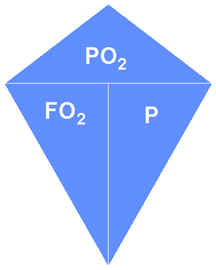Formulae

VO2 = (Qs (FsO2 - FiO2 )) /  1 - FiO2

FiO2 = ((FsO2 * Qs) - VO2) /  Qs - VO2

VO2 = Oxygen metabolic rate

FiO2 = Inspired oxygen

Qs = Flow rate in lpm

FsO2 = supply oxygen percentage

Equivalent Air Depth

EAD = ((FN2 / .79) * D / 33) - 33

FN2 - Fraction of Nitrogen

D - Depth

Memory Aid for;                                    Pg = P * Fg         P = Pg / Fg         Fg = Pg / PPg - Partial Pressure of the gas i.e. PO2

P - Pressure in ata

Fg - Fraction of the gas i.e. FO2

Ambient pressure

( P / 33 ) + 1

P - Pressure in ata

Flow through a sonic orifice

Flow  (lpm) = 11Pbar * D2

P = pressure in bars

D = diameter in mm